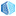# Modeling

This category contains 6 nodes.

##### AutoQSAR Build Model

Generate QSAR models for a chosen property of a set of compounds, and apply the QSAR model to other compounds.

##### AutoQSAR Predict

Choose structures to make predictions for and the model to apply.

##### Bayes Classification Model Building

Build a Bayes model from binary or continuous training data to predict the probability of a molecule at each activity level.

##### Bayes Classification Prediction

Test a previously built Bayes model

##### PLS Model Building

Build a multiple linear regression model based on variables derived from a PLS (Partial Least-Squares) analysis of a selection of properties for a […]

##### PLS Prediction

Test a previously built PLS (Partial Least-Squares) regression model